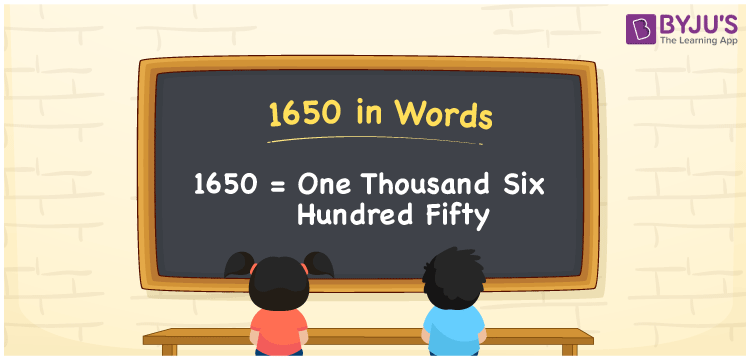# 1650 in Words

1650 in words is written as One thousand six hundred fifty. In both the International System of Numerals and the Indian System of Numerals, 1650 is written as One thousand six hundred fifty.

We often use 1650 as “1650 students in the assembly hall”. 1650 represents a quantity, “how much of something” or “how many of something”. Hence, the number 1650 is a Cardinal Number.

 1650 in words One thousand six hundred fifty One thousand six hundred fifty in Number 1650

## 1650 in English Words

1650 in English words is read as “One thousand six hundred fifty.”## How to Write 1650 in Words?

To write 1650 in words, we shall use the place value chart. In the place value chart, put 1 in the thousands, 6 in the hundreds, 5 in the tens and 0 in the ones, respectively. Let us make a place value chart to write the number 1650 in words.

 Thousands Hundreds Tens Ones 1 6 5 0

Thus, we can write the expanded form as

1 × Thousand + 6 × Hundred + 5 × Ten + 0 × One

= 1 × 1000 + 6 × 100 + 5 × 10 + 0 × 1

= 1000 + 600 + 50 + 0

= 1650

= One thousand six hundred fifty.

1650 is a natural number which lies between1649 and 1651.

1650 in words – One thousand six hundred fifty

• Is 1650 an odd number? – No
• Is 1650 an even number? – Yes
• Is 1650 a perfect square number? – No
• Is 1650 a perfect cube number? – No
• Is 1650 a prime number? – No
• Is 1650 a composite number? – Yes

## Frequently Asked Questions on 1650 in Words

Q1

### How to write 1650 in words?

1650 in words is written as One thousand six hundred fifty.
Q2

### How to write 1650 in words in the International and Indian System of Numerals?

In both, the system of numerals, 1650 in words, is written as One thousand six hundred fifty.
Q3

### How to write 1650 in a place value chart?

In the place value chart, write 1 in the thousands, 6 in the hundreds, 5 in the tens and 0 in the ones, respectively.